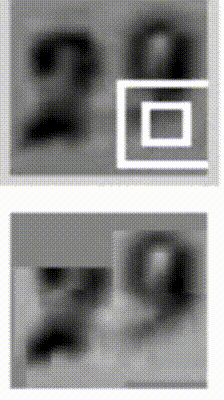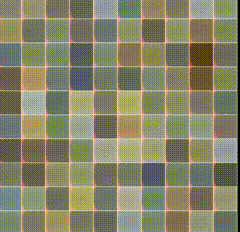# Week 5: Sequence to Sequence

[reveal]

Abstract:

This lecture will continue on RNNs and their evolution as methods for performing sequence to sequence.

### RNN: Recap### The state update rule: naive

$\mathbf{h}_{t+1} = \phi(W_h \mathbf{h}_t + W_x \mathbf{x}_t + \mathbf{b_h})$

### The state update rule: GRU

\begin{align} \mathbf{h}_{t+1} &= \mathbf{z}_t \odot \mathbf{h}_t + (1 - \mathbf{z}_t) \odot \tilde{\mathbf{h}}_t \\ \tilde{\mathbf{h}}_t &= \phi\left(W\mathbf{x}_t + U(\mathbf{r}_t \odot \mathbf{h}_t)\right)\\ \mathbf{r}_t &= \sigma(W_r\mathbf{x}_t + U_r\mathbf{h}_t)\\ \mathbf{z}_t &= \sigma(W_z\mathbf{x}_t + U_z\mathbf{h}_t)\\ \end{align}

### implementing branching logic

…in code:

if r:
return 5
else:
return 3

…in algebra:

return r*5 + (1-r)*3

### The state update rule: GRU

\begin{align} \mathbf{h}_{t+1} &= \mathbf{z}_t \odot \mathbf{h}_t + (1 - \mathbf{z}_t) \odot \tilde{\mathbf{h}}_t \\ \tilde{\mathbf{h}}_t &= \phi\left(W\mathbf{x}_t + U(\mathbf{r}_t \odot \mathbf{h}_t)\right)\\ \mathbf{r}_t &= \sigma(W_r\mathbf{x}_t + U_r\mathbf{h}_t)\\ \mathbf{z}_t &= \sigma(W_z\mathbf{x}_t + U_z\mathbf{h}_t)\\ \end{align}

### Side note: dealing with depth### Side note: dealing with depth### Very deep networks are hard to train

• their performance degrades with depth
• VGG19: 19-layer ConvNet

### Deep Residual Networks (ResNets)### Deep Residual Networks (ResNets)### ResNets

• allow for much deeper networks (101, 152 layer)
• performance increases with depth
• new record in benchmarks (ImageNet, COCO)
• used almost everywhere now

### Resnets behave like ensemblesfrom (Veit et al, 2016)

### DenseNets### Back to RNNs

• like ResNets, LSTMs create “shortcuts”
• allows information to skip processing
• data-dependent gating
• data-dependent shortcuts

### Visualising RNN behaviours

See this distill post

### RNN: different usesfigure from Andrej Karpathy’s blog post

### RNNs for images### RNNs for images### RNNs for painting### RNNs for painting### Spatial LSTMs### Spatial LSTMs generating textures### Seq2Seq: sequence-to-sequence### Seq2Seq: neural machine translation### Show and Tell: “Image2Seq”### Show and Tell: “Image2Seq”### Sentence to Parsing tree “Seq2Tree”### General algorithms as Seq2Seq

travelling salesman### General algorithms as Seq2Seq

convex hull and triangulation### Pointer networks### Revisiting the basic idea### Attention layer### Attention layer

Attention weights:

$\alpha_{t,s} = \frac{e^{\mathbf{e}^T_t \mathbf{d}_s}}{\sum_u e^{\mathbf{e}^T_t \mathbf{d}_s}}$

Context vector:

$\mathbf{c}_s = \sum_{t=1}^T \alpha_{t,s} \mathbf{e}_t$

### Attention layer visualised

{### To engage with this material at home

Try the char-RNN Exercise from Udacity.

• neural machine translation (historical note)
• image captioning: encoder is a CNN, decoder is RNN
• forgetting problem revisited
• asking the network too much
• allowing the decoder to look back at encoder states
• pointer networks